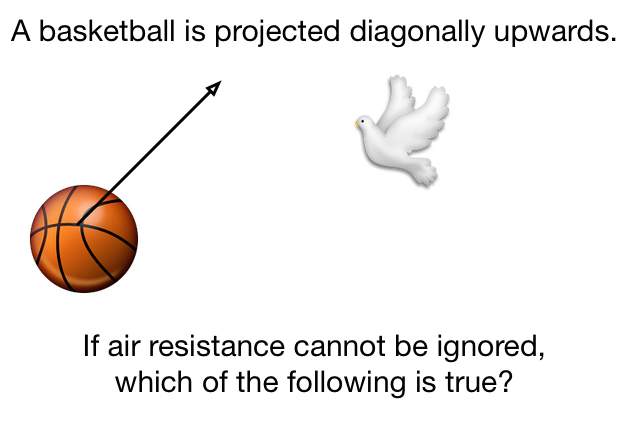# Projectile Motion With Air ResistanceWhich of the following statements are true for a basketball that is projected diagonally upwards (air resistance cannot be ignored)?

a. The basketball's kinetic energy is zero at the highest point of its motion.

b. The basketball’s average kinetic energy is smaller when traveling upwards than downwards.

c. The time taken for the basketball to travel upwards is less than the time taken to travel downward.

d. The horizontal distance traveled when the basketball is moving upwards is equal to the horizontal distance traveled when the basketball is moving downwards.

×